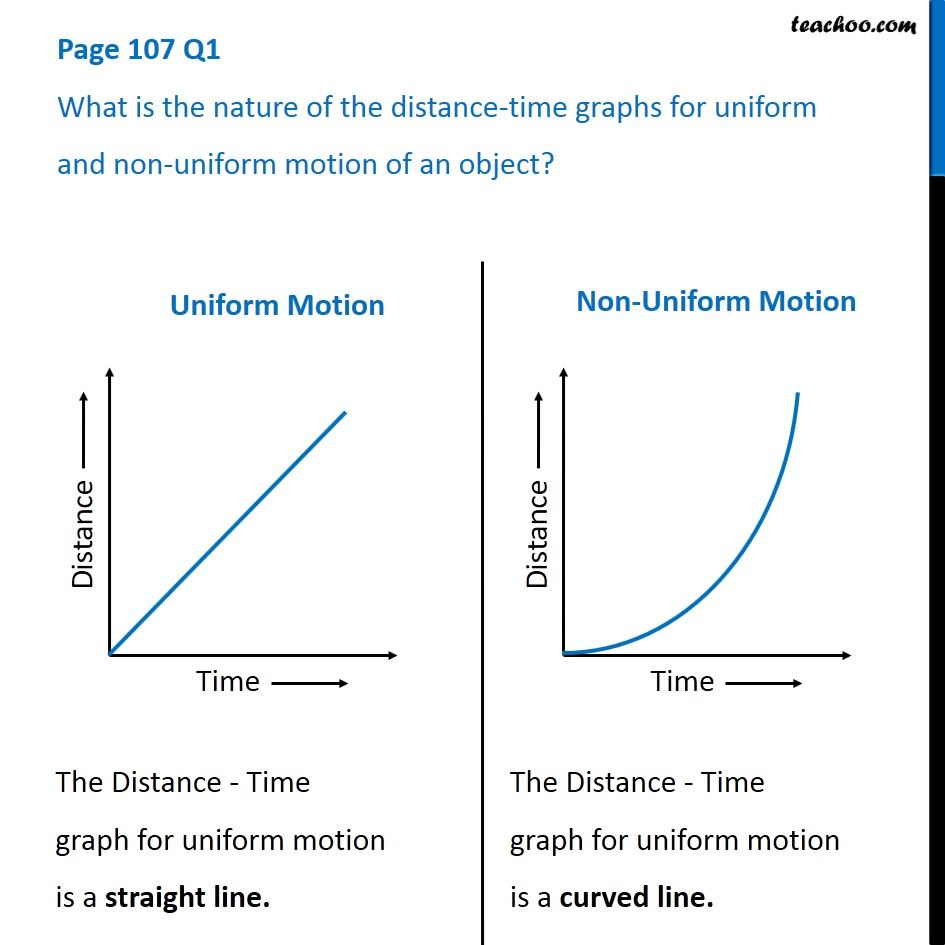1. Class 9
2. Chapter 8 Class 9 - Motion (Term 1)
3. Questions from Inside the chapter

Transcript

Page 107 Q1 What is the nature of the distance-time graphs for uniform and non-uniform motion of an object? Uniform Motion The Distance - Time graph for uniform motion is a straight line. Non-Uniform Motion The Distance - Time graph for uniform motion is a curved line.

Questions from Inside the chapter

Class 9
Chapter 8 Class 9 - Motion (Term 1)